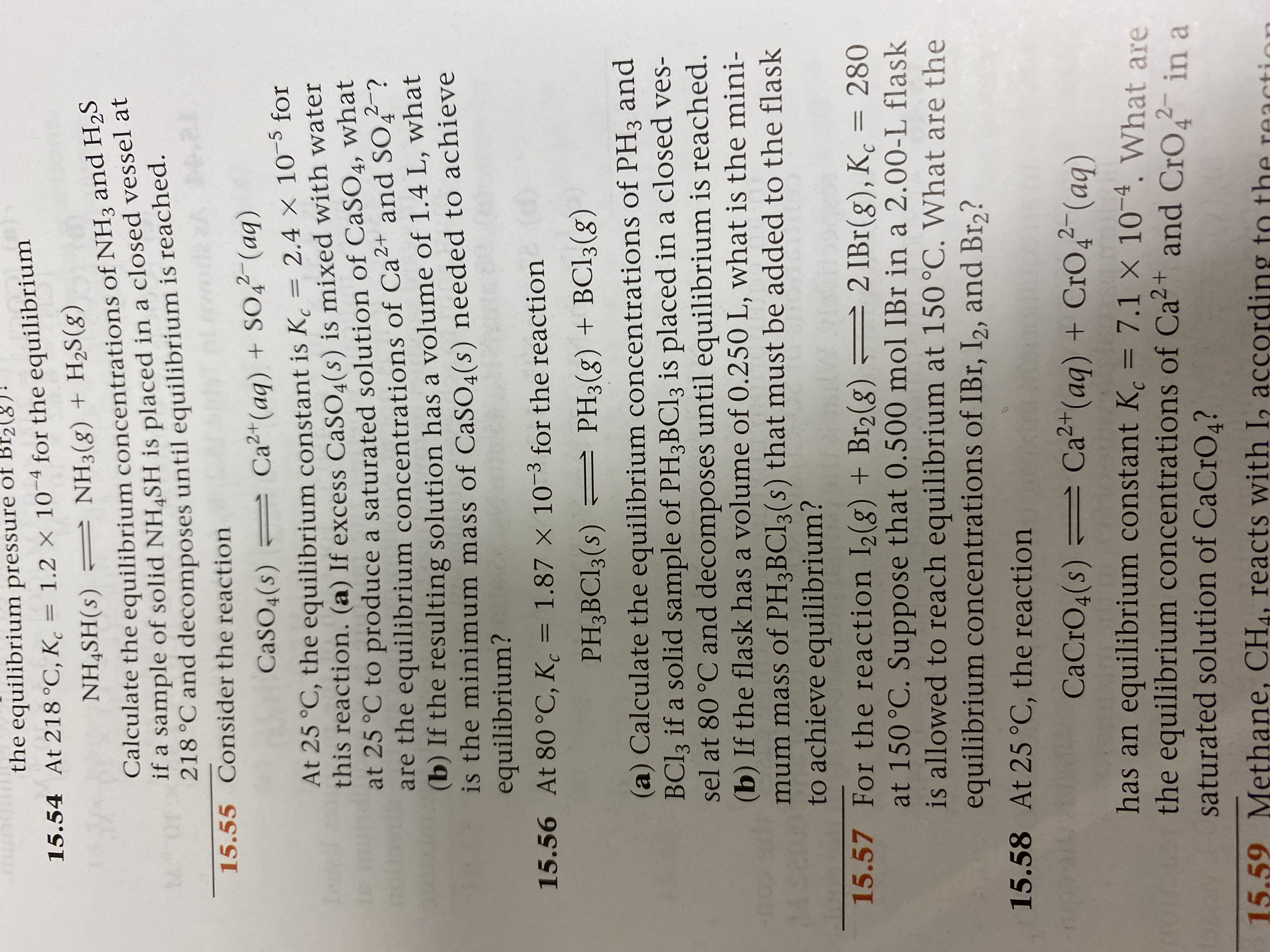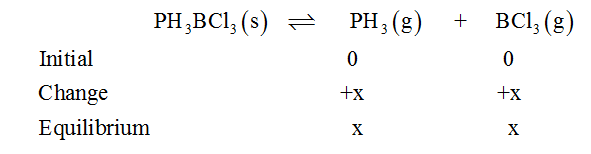# the equilibrium pressure of Bf2(8)15.54 At 218 °C, K̟ = 1.2 × 10-4 for the equilibriumNH4SH(s) = NH3(g) + H2S(g)Calculate the equilibrium concentrations of NH3 and H2Sif a sample of solid NH SH is placed in a closed vessel at218 °C and decomposes until equilibrium is reached.15.55 Consider the reactionCaSO4(s) = Ca2+(aq) + SO,² (aq)At 25 °C, the equilibrium constant is K, = 2.4 × 10¯³ forthis reaction. (a) If excess CASO.(s) is mixed with waterat 25 °C to produce a saturated solution of CaSO4, whatare the equilibrium concentrations of Ca²+ and SO7?(b) If the resulting solution has a volume of 1.4 L, whatis the minimum mass of CASO.(s) needed to achieveequilibrium?4.15.56 At 80 °C, K̟ = 1.87 × 10-3 for the reaction%3DPH3BCI3(s) = PH3(g) + BC13(g)(a) Calculate the equilibrium concentrations of PH3 andBCI3 if a solid sample of PH3BC13 is placed in a closed ves-sel at 80 °C and decomposes until equilibrium is reached.(b) If the flask has a volume of 0.250 L, what is the mini-mum mass of PH3BC13(s) that must be added to the flaskto achieve equilibrium?15.57 For the reaction I2(g) + Br2(g)2 IBr(g), K. = 280at 150 °C. Suppose that 0.500 mol IBr in a 2.00-L flaskis allowed to reach equilibrium at 150°C. What are theequilibrium concentrations of IBr, I2, and Br,?15.58 At 25 °C, the reactionCaCrO4(s) Ca2(aq)+ CrO2 (aq)has an equilibrium constant K = 7.1 × 10-4. What arethe equilibrium concentrations of Ca?+saturated solution of CaCrO4?%3Dooand CrO,2- in a415.59 Methane, CH4, reacts with I, according to the reactio

Question
1 views

Question 15.56 part A onlyhelp_outlineImage Transcriptionclosethe equilibrium pressure of Bf2(8) 15.54 At 218 °C, K̟ = 1.2 × 10-4 for the equilibrium NH4SH(s) = NH3(g) + H2S(g) Calculate the equilibrium concentrations of NH3 and H2S if a sample of solid NH SH is placed in a closed vessel at 218 °C and decomposes until equilibrium is reached. 15.55 Consider the reaction CaSO4(s) = Ca2+(aq) + SO,² (aq) At 25 °C, the equilibrium constant is K, = 2.4 × 10¯³ for this reaction. (a) If excess CASO.(s) is mixed with water at 25 °C to produce a saturated solution of CaSO4, what are the equilibrium concentrations of Ca²+ and SO7? (b) If the resulting solution has a volume of 1.4 L, what is the minimum mass of CASO.(s) needed to achieve equilibrium? 4. 15.56 At 80 °C, K̟ = 1.87 × 10-3 for the reaction %3D PH3BCI3(s) = PH3(g) + BC13(g) (a) Calculate the equilibrium concentrations of PH3 and BCI3 if a solid sample of PH3BC13 is placed in a closed ves- sel at 80 °C and decomposes until equilibrium is reached. (b) If the flask has a volume of 0.250 L, what is the mini- mum mass of PH3BC13(s) that must be added to the flask to achieve equilibrium? 15.57 For the reaction I2(g) + Br2(g) 2 IBr(g), K. = 280 at 150 °C. Suppose that 0.500 mol IBr in a 2.00-L flask is allowed to reach equilibrium at 150°C. What are the equilibrium concentrations of IBr, I2, and Br,? 15.58 At 25 °C, the reaction CaCrO4(s) Ca2 (aq)+ CrO2 (aq) has an equilibrium constant K = 7.1 × 10-4. What are the equilibrium concentrations of Ca?+ saturated solution of CaCrO4? %3D oo and CrO,2- in a 4 15.59 Methane, CH4, reacts with I, according to the reactio fullscreen
check_circle

Step 1

The ICE table for the chemical reaction can be drawn as follows-...

### Want to see the full answer?

See Solution

#### Want to see this answer and more?

Solutions are written by subject experts who are available 24/7. Questions are typically answered within 1 hour.*

See Solution
*Response times may vary by subject and question.
Tagged in

### Chemistry